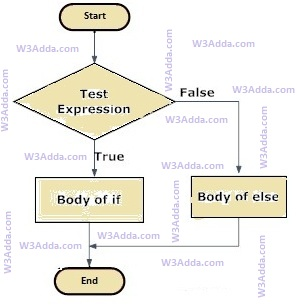# C If Else Statement

In this tutorial you will learn about the C If Else Statement and its application with practical example.

## C if-else Statement

The c if-else statement is an extended version of the If statement. When we want to execute a block of code when the if the condition is true and another block of code when the if the condition is false, In such a case we use the if-else statement. The statements inside the “if” body only execute if the given condition returns true. If the condition returns false then the statements inside the “else” body are executed.

But what if we want to do something else if the condition is false. Here comes the C else statement. We can use the else statement with the if statement to execute a block of code when the condition is false.

### C If else Statement flowchart### if-else Statement Syntax

The general syntax of if-else statement is as follows:

Syntax:-

### Working of if-else Statement

Here, the if statement evaluates the test condition inside the parenthesis `()`. The Condition is a Boolean expression that results in either True or False. if it results in True then statements inside if the body is executed, if it results in False then statements inside else body is executed.

### C if-else Example

Below is a simple example to demonstrate the use of if-else in c programming:

Example:-

Output:-In this tutorial we have learn about the C If Else Statement and its application with practical example. I hope you will like this tutorial.